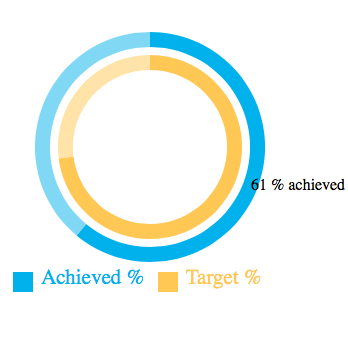## Concentric radial progress indicators with d3js

Thu 08 December 2016

### The result

This will be more of a cookbook, with some rationale and explanations sprinkled in rather than a full on ground-up introduction to d3js (otherwise it would never get off the ground!).

Here's one I prepared earlier, you can imagine it's like some sort of fitbit or other health app where you're aiming for some percentage or value and you have a target. In this it's a radial progress graph meaning the outside and inside rings shows the value between 0 and 100%, and the rest of the value to 100% is more faded if the value is less than 100%.### The recipe

Here's the HTML that holds the javascript.

```<!DOCTYPE html>
<html>
<meta content="text/html; charset=utf-8" http-equiv="Content-type">
<title>Chart Test</title>
<script src="https://d3js.org/d3.v4.min.js"></script>
</script>
<style type="text/css">
svg {
overflow: visible !important;
}
</style>
<body>
<script type="text/javascript">
... (see below)
</script>
</body>
</html>
```

First we setup our helper and variables

```function rgba(rgb, a) {
return "rgba(" + rgb + "," + rgb + "," + rgb + "," + a + ")";
}
var data = [["Achieved", 61, 'blue'],["Target", 73, 'red']],
w = 290, h = 290,
ringWidth = 15, pad = 8, textBox = 20,
radius = (Math.min(w, h) / 2) - textBox / 2.0;
ringColours = [[0, 174, 239],[255, 202, 58]],
svg = d3.select("body").append("svg").attr('width', w).attr('height', h).append(
'g').attr('transform', 'translate(' + (w / 2) + ',' + (h / 2) + ')');
```

Now that we have a bare SVG element, let's start the graphing, by looping over our data to be graphed as rings and drawing them concentrically. (we'll cover the method definiton afterwards).

```data.forEach(function (e, i) {
makeCircle(e, i)
});
```

Now that the graph has been drawn we can put the legend in, we'll come back and explain how the circles works.

```var legend = svg.selectAll('.legend').data(data).enter().append('g').attr(
'class', 'legend').attr('transform', function (d, i) {
return 'translate(' + (pad - w / 2 + i * w / 2) + ',' + (h / 2 - textBox) + ')';
});
legend.append('rect').attr('width', textBox).attr('height', textBox).style(
'fill',
function (d, i) {
return rgba(ringColours[i], 1.0);
}).style('stroke', d3.category20);
'fill',
function (d, i) {
return rgba(ringColours[i], 1.0);
}).attr('font-size', "1.3em").text(function (d, i) {
return d + " %";
});
```

Here's the function that can make concentric circles, it starts by calculating the outer radius `out` and providing the ringWidth. There's mouse-over information provided also.

```function makeCircle(item, level) {
rData = [{
"on": true,
"val": item
}, {
"on": false,
"val": 100 - item
}];
var pie = d3.pie().sort(null).value(function (d) {
return d.val;
});
var out = radius - (textBox / 2 + 10 + (level * (ringWidth + pad)))
var g = svg.selectAll(".arc").data(pie(rData)).enter().append("g");
g.append("path").attr("d", arc).style("fill", function (d) {
return rgba(ringColours[level], d.data.on ? 1.0 : 0.5);
}).on("mouseover", function () {
d3.select(this.parentNode).select("text").style("display", "block");
}).on("mouseout", function () {
d3.select(this.parentNode).select("text").style("display", "none");
});
var text = g.append("text").attr("transform", function (d) {
return "translate(" + arc.centroid(d) + ")";
}).style("display", "none").attr("dy", ".35em").text(function (d, i) {
return rData[i].val + " % " + (d.data.on ? "" : "not ") + "achieved";
});
}
```

Unfortunately there's alot of chaining commands needed as is the javascript way. Hopefully this is a starting point for some awesome types of things that can be done with d3js.